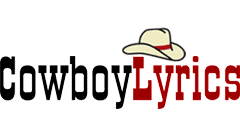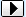MENU Request Lyrics   Add/Correct Lyrics   Links Page   Contact Us   Privacy Policy   DMCA Policy MERCHANDISE Country CDs   Country Sheet MusicBROWSE LYRICS AND TABS BY ARTIST/BAND NAME:SEARCH:A B C D E F G H I J K L M N O P Q R S T U V W X Y Z ## Set Me Free 2 chords - Kelly Reckless```Set Me Free

~^~^~^~^~^~^~^~^~^~^~^~^~^~^~^~^~^~^~^~^~^~^~^~^~^~^~^~^~^~^~^~^~^~^~^~^
G
Set me free, set me free,
C                  G
I need a little more room to breathe
D                    C
And the freedom to do what I please.
G
Mama, set me free.
G
Set me free, set me free.
C              G
Let me see what I can see.
D                  C
Have mercy on a boy like me,
G
Mama, set me free.

D                  C              G
Drop me off on a mountain top somewhere.
D                C                G
I'll point them down and say my prayers.
D             C         G
No one understands it, maybe they don't care.
D           C                  G
We're only here because it's there.

G
Set me free, set me free.
C             G
I wanna be what I can be.
D                    C
City livin' ain't livin' to me,
G
Mama, set me free.
G
Set me free, set me free.
C             G
Let me see what I can be
D                 C
Have mercy on a boy like me
G
Mama, set me free.

G   C  G   D  C  G  2X

D              C           G
I'll go where people don't go.
D                C           G
Where the iron horses don't roll.
D               C            G
High above the sea of grey below
D              C                          G
And the great mystery and the cold wind blows.

G
Set me free, set me free
C                   G
I need a little more room to breathe
D                    C
And the freedom to do what I please,
G
Mama, set me free.
G
Set me free, set me free,
C             G
Let me see what I can be.
D                   C
Have mercy on a boy like me,
G
Mama, set me free.
D                   C
Have mercy on a boy like me,
G
Mama, set me free.
D                   C
Have mercy on a boy like me,
G
Mama, set me free.

D  C  G  D  C  G
G  C  G  D  C  G 4X
D  C  G  D  C  G  D  C  G  D  C  G

~^~^~^~^~^~^~^~^~^~^~^~^~^~^~^~^~^~^~^~^~^~^~^~^~^~^~^~^~^~^~^~^~^~^~^~^
CHORDS
D    =   X00232
C    =   X32010
G    =   320033

enjoy :)
```

### Kelly Reckless Chords • Kelly Reckless Lyrics